### 3.1.5 From Regular Expressions to FSAs

Proof, that there is a FSA for every regular expression.

We now prove one direction of the equivalence between regular expressions and FSAs: We will show that there is a FSA for every regular expression. The nice thing about this proof is that it is contructive - it proceeds by showing us what such an automaton will actually look like.

Our proof is by induction over the number of operators (that is, occurences of concatenation,and) in a regular expression. We first show that there are automata for all possible singleton regular expressions. Then we assume that there are automata for the regular expressions with less thanoperators, and show that there is one for each regular expression with n operators.

Basis

We first have to look at regular expressions without any operators. This means, we are talking about,orfor some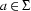. Forwe can use the following automaton: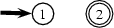Obviously, we can never get to the final state in this automaton, therefore no word is accepted.

For, the following automaton can be given: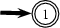In this automaton we are at the final state at the moment we begin our computation (and we cannot get out of it). Therefore the automaton accepts only ``input'' having no symbol (that is: the empty word).

We've already seen (in Section 3.1.2) the pattern of how to construct automata forfor any.

Induction

Now let's turn to complex regular expressions, i.e. ones that do contain operators. More precisely, we assume that there are automata for the regular expressions with fewer thanoperators (with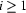), and take a regular expressionwithoperators. We have to look at three cases.

1. Concatenation: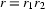2. Union: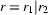3. Kleene Closure: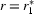In all three cases,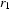and (where applicable)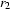have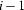operators. Therefore there are FSAs for them by our induction hypothesis. We now have to build an automaton foron the basis of these given automata. All we have to do is make a few additions according to the additional operator used in. We will basically apply the ideas already seen in Section 3.1.2.

Let's go through the cases one by one. We assume that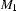is an automaton for,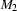corresponds to, and that the states inare disjoint from those in(we can always achieve this by renaming):

Concatenation ():

We construct an automaton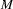that consists ofandin series. That is,will accept a word by runningon the first part, then jumping toand running it on the second part of the word.will look like this: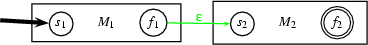The start state ofis also the start state of, and the final state ofis also the final state of. The final state ofand the start state ofhave become ``normal'' states, but are now connected by a jump arc. Formally (where indices 1 and 2 indicate that an entity originally belongs toor):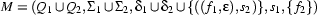Union ():

We construct an automatonthat consists ofandin parallel. That is,will accept a word by jumping into eitheror, running it on the word, and then jumping to a new final state.will look like this: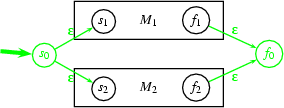The new start state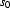is connected by jump arcs to the former start states ofand. Thus it serves as the common start state for going throughor. Similarly, the former final states ofandare now both connected to a new final state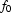by jump arcs. This new final state serves as the common final state for going throughor. Formally, the new automaton looks like this: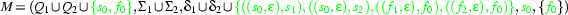Kleene Closure (:

We build a new automatonthat ``embeds''. Inwe can either bypassand jump directly from the start state to the final state, or loop by jumping into, going through, and then jumping back to the (former) start state of. After looping an arbitrary number of times on, we can jump to the final state. Thusaccepts(via the bypassing jump arc) as well as all combinations of words that are accepted by(by looping on the-part of). Here's the picture: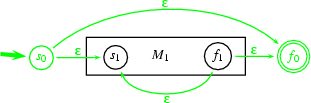We had to add two states (the new start and final statesand), and four jump edges (one into, one out of, one to bypass it and one to loop). Thus this is the formal characterization of the new automaton: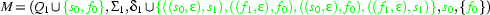So we've shown that for any regular expression there is an FSA that characterizes the same language. And in fact we've also shown how to construct such an automaton from any given regular expression. We just have to formulate a recursive procedure based on our inductive proof. We won't give an implementation that builds FSAs based on regular expressions here. But parts of the proof we've just seen will be very useful in Section 3.3, where we implement a tool for performing operations on given FSAs.

Kristina Striegnitz, Patrick Blackburn, Katrin Erk, Stephan Walter, Aljoscha Burchardt and Dimitra Tsovaltzi
Version 1.2.5 (20030212)